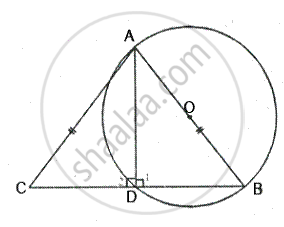Share

Prove that the Circle Drawn on Any One of the Equal Sides of an Isosceles Triangle as Diameter Bisects the Base. - ICSE Class 10 - Mathematics

ConceptArc and Chord Properties - Angle in a Semi-circle is a Right Angle

Question

Prove that the circle drawn on any one of the equal sides of an isosceles triangle as diameter bisects the base.

SolutionGiven – In ∆ABC, AB = AC and a circle with AB as diameter is drawn
Which intersects the side BC and D.
To prove – D is the mid point of BC

Construction – Join AD.
Proof - ∠1 = 90°     [Angle in a semi circle]
But ∠1+ ∠2 = 180°       [Linear pair]

∴ ∠2 = 90°
Now in right ∆ABD and ∆ACD, Hyp. AB = Hyp. AC [Given]
∴ By the right Angle – Hypotenuse – side criterion of congruence, we have

ΔABD ≅ ∆ACD         [ RHS criterion of congruence]

The corresponding parts of the congruent triangle are congruent.
∴ BD = DC      [c.p.c.t]
Hence D is the mid point of BC.

Is there an error in this question or solution?

Video TutorialsVIEW ALL 

Solution Prove that the Circle Drawn on Any One of the Equal Sides of an Isosceles Triangle as Diameter Bisects the Base. Concept: Arc and Chord Properties - Angle in a Semi-circle is a Right Angle.
S